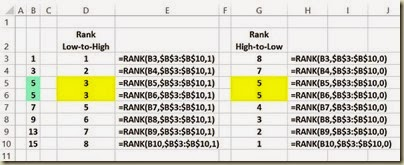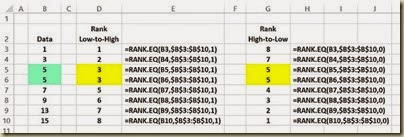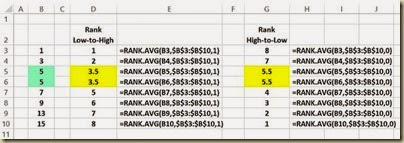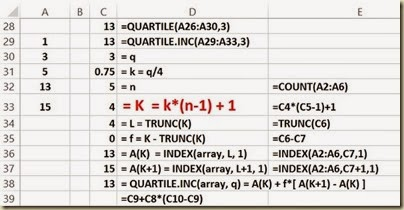# Simplifying Excel Ranking Functions: RANK(), RANK.AVG(), RANK.EQ(), PERCENTILE(), PERCENTILE.INC(), PERCENTILE.EXC(), QUARTILE(), QUARTILE.INC (), QUARTILE.EXC()

## Simplifying RANK(), RANK.EQ(), and RANK.AVG()

Ranking functions RANK(), RANK.EQ(), and RANK.AVG() return the rank of a data value within a data set. Prior to Excel 2010 RANK() was the sole ranking function. In Excel 2010 and beyond RANK() will still work but its functionality has been duplicated by a new ranking function RANK.EQ(). In addition, another useful ranking function RANK.AVG() has been added.

The format of each of these functions is as follows:

RANK(value to be ranked, data range, [order])

RANK.EQ(value to be ranked, data range, [order])

RANK.AVG(value to be ranked, data range, [order])

The value to be ranked is a specific number or value is a data set that will be ranked. The data range represents the set of values that will provide the basis for the ranking. The order is an optional value. Setting the order to 0 or leaving it blank will rank data in descending order, i.e., the highest value in the data range will be assigned a rank = 1. Setting the order to 1 will rank data in ascending order, i.e., the lowest value in the data range will be assigned a rank = 1.

The difference between RANK.EQ() and RANK.AVG() is how tied data values within the data set are ranked. RANK.EQ() assigns the lowest rank of the tied values to all of the tied values. RANK.AVG() assigns the average rank of the tied values to all of the tied values.

RANK.AVG() is useful when performing certain nonparametric tests in Excel. Several nonparametric tests require that data be ranked with tied data values being assigned the average rank of the tied values as is done by RANK.AVG(). These nonparametric tests include the Mann-Whitney U Test, the Wilcoxon Signed-Rank Test, and the Friedman Test.

The functionality of the RANK() function is shown as follows. The raw data that is being ranked has already been sorted in order to more easily convey the functionality of the RANK() function.(Click On Image To See a Larger Version)

The functionality of the RANK.EQ() function is shown in the following diagram. RANK.EQ() is equivalent to RANK() and should now be used in its place. Note that tied data values are all assigned the lowest rank of the tied values. The raw data that is being ranked has already been sorted in order to more easily convey the functionality of the RANK.EQ() function.(Click On Image To See a Larger Version)

The functionality of the RANK.AVG() function is shown in the following diagram. Note that tied data values are all assigned the average rank of the tied values. There is no equivalent to this function in versions of Excel prior to 2010. The raw data that is being ranked has already been sorted in order to more easily convey the functionality of the RANK.AVG() function.(Click On Image To See a Larger Version)

## Simplifying PERCENTILE(), PERCENTILE.INC(), and PERCENTILE.EXC()

Percentile functions PERCENTILE(), PERCENTILE.INC(), and PERCENTILE.EXC() return the value at a given percentile in relation to a given data set. For example, given the data set {1, 3, 5, 13, 15} in cells A2:A6, PERCENTILE(A2:A6,0.3) = 3.4. This means that the value 3.4 occupies the 30th percentile of the given data set.

An example of the use of the percentile functions would be to determine which test scores within a group of test score are at or above the 95th percentile of the group. The percentile functions would return that value that would occupy that 95th percentile of the group.

The percentile functions are typically used to establish a threshold of acceptance or failure.

Prior to Excel 2010 PERCENTILE() was the sole percentile function. In Excel 2010 and beyond PERCENTILE() will still work but its functionality has been duplicated by a new percentile function PERCENTILE.INC(). In addition, another percentile function PERCENTILE.EXC() has been added.

The format of each of these functions is as follows:

PERCENTILE(data range, percentile)

PERCENTILE.INC(data range, percentile)

PERCENTILE.EXC(data range, percentile)

The percentile can be any number between 0 and 1.

The value to be ranked is a specific number or value is a data set that will be ranked. The data range represents the set of values that will provide the basis for the ranking. The order is an optional value. Setting the order to 0 or leaving it blank will rank data in descending order, i.e., the highest value in the data range will be assigned a rank = 1. Setting the order to 1 will rank data in ascending order, i.e., the lowest value in the data range will be assigned a rank = 1.

Both PERCENTILE.EXC() and PERCENTILE.INC() and its equivalent PERCENTILE() first rank the values in the given data set from 1 (assigned to the lowest value in the data set) to n (assigned to the highest value in the data set). The rank K is then calculated. The main difference between is the method of calculating K as shown in the following Excel algorithms for these functions. If K is not an integer value, linear interpolation between the nearest values in the data set to determine the value that would occupy the given percentile.

Another difference between the percentile functions is that PERCENTILE.EXC only works if k is between 1/n and 1-1/n, where n is the number of elements in array. PERCENTILE.INC works for any value of k between 0 and 1.

The Excel algorithm for equivalent functions PERCENTILE() and the equivalent PERCENTILE.INC() is as follows:

The Excel algorithm for PERCENTILE.EXC() is as follows:

## Simplifying PERCENTRANK(), PERCENTRANK.INC(), and PERCENTRANK.EXC()

PERCENTRANK(), PERCENTRANK.INC(), and PERCENTRANK.EXC() are the inverse functions of PERCENTILE(), PERCENTILE.INC(), and PERCENTILE.EXC().

PERCENTRANK(), PERCENTRANK.INC(), and PERCENTRANK.EXC() return the percentile that a given data value has in relation to set of data values.

PERCENTILE(), PERCENTILE.INC(), and PERCENTILE.EXC() return the data value that would occur at a given percentile in relation to a set of data values.

The following diagram shows the relationship of the PERCENTRANK functions to the PERCENTILE functions. In each of these cases the percentile is the 30th percentile.

## Simplifying QUARTILE(), QUARTILE,INC(), and QUARTILE.EXC()

The quartile functions are special cases of the percentile functions. The equivalent functions are as follows:

QUARTILE(Data range, 0) = PERCENTILE(Data range, 0) = MIN(Data range)

QUARTILE(Data range, 1) = PERCENTILE(Data range, .25)

QUARTILE(Data range, 2) = PERCENTILE(Data range, .5) = MEDIAN(Data range)

QUARTILE(Data range, 3) = PERCENTILE(Data range, .75)

QUARTILE(Data range, 4) = PERCENTILE(Data range, 1) = MAX(Data range)

QUARTILE.INC(Data range, 0) = PERCENTILE.INC(Data range, 0)

QUARTILE.INC(Data range, 1) = PERCENTILE.INC(Data range, .25)

QUARTILE.INC(Data range, 2) = PERCENTILE.INC(Data range, .5)

QUARTILE.INC(Data range, 3) = PERCENTILE.INC(Data range, .75)

QUARTILE.INC(Data range, 4) = PERCENTILE.INC(Data range, 1)

QUARTILE.EXC(Data range, 0) = PERCENTILE.EXC(Data range, 0)

QUARTILE.EXC(Data range, 1) = PERCENTILE.EXC(Data range, .25)

QUARTILE.EXC(Data range, 2) = PERCENTILE.EXC(Data range, .5)

QUARTILE.EXC(Data range, 3) = PERCENTILE.EXC(Data range, .75)

QUARTILE.EXC(Data range, 4) = PERCENTILE.EXC(Data range, 1)

The Excel algorithm for equivalent functions QUARTILE() and the equivalent QUARTILE.INC() is as follows:(Click On Image To See a Larger Version)

The Excel algorithm for QUARTILE.EXC() is as follows:

Excel Master Series Blog Directory

Statistical Topics And Articles In

This Blog

You Will Become an Excel Statistical Master!

1.easy excel rank
thanks

2.Not really much of a formula user in excel because I only know the basics. But with these tips on how to simplifying excel ranking functions, it will be more easy for me to generate reports. Glad I came across this view original source.

3.Great post! I hope it will be useful for me as I don't wan to pay someone to write my research paper. I'd rather research and write by myself.

4.Similarly, knowing which areas of physics you need help with will help you to find a tutor who is qualified. Overall, the most important thing to consider when searching for a physics tutor, is someone who is flexible in their tutoring style and willing to try new teaching techniques that best fit their student.

Physics assignments are complicated projects that require a lot of time, due to the use of Physics formulas and the prerequisites in math. Completing the physics assignments successfully requires a great deal of knowledge, skills and sometimes assistance from qualified online physics help providers.You can get professional homework help at How Homework Was Invented

5.Thanks for the blog.Thanks Again. Awesome Androdumpper Whatsdog Testdpc

6.Your substance is out and out splendid from multiple points of view. I think this is connecting with and enlightening material. Much obliged to you such a great amount for thinking about your substance and your perusers. blog comments

7.This is a brilliant blog! I'm very happy with the comments!.. posizionamento nei motori di ricerca

8.Thanks for taking the time to discuss this, I feel strongly about it and love learning more on this topic. If possible, as you gain expertise, would you mind updating your blog with more information? It is extremely helpful for me.
pikdo

9.The effectiveness of IEEE Project Domains depends very much on the situation in which they are applied. In order to further improve IEEE Final Year Project Domains practices we need to explicitly describe and utilise our knowledge about software domains of software engineering Final Year Project Domains for CSE technologies. This paper suggests a modelling formalism for supporting systematic reuse of software engineering technologies during planning of software projects and improvement programmes in Final Year Project Centers in Chennai.

Spring Framework has already made serious inroads as an integrated technology stack for building user-facing applications. Spring Framework Corporate TRaining the authors explore the idea of using Java in Big Data platforms.
Specifically, Spring Framework provides various tasks are geared around preparing data for further analysis and visualization. Spring Training in Chennai

10.Thank you for sharing information. Wonderful blog & good post.
Sony WALKMAN App

11.12.13.Thanks for sharing it is important for me. I also searched for that from here. Visit our site AVG belgie

14.That’s a nice article, thank you for a great article. It helped me a lot. Keep it up Must Visit Epson Printer nummer belgie

15.Hi this post is very nice.finally i got all those information what i really wanted to know. thankyou for sharing this usefull information.here i want to share the information about mcafee security. as we all have been digitalied and we do multiple works together so if you face any problem or need any assist about MCAFEE so pleae visit over this site-mcafee Antivirus technische ondersteuning

16.Hi your post is really appreciatable.I really thankful for this post .here i would llike to share some information about HP Printer.We can resolve the smallest problem on same time If you are facing any problem relate to your HP Printer's so this is the right place to get smallest information about HP PRINTER for the further information please visit on our website- hp printer technische ondersteuning

17.Hi thankyou for this great helpful information.this information is really useful at today's time.you had easily explained whole information in a short note.now i want to share some information about avast antivirus.So if you are facing any problem regarding Avast so you can simply visit on our site-avast technische ondersteuning

18.Hi your post is very nice and i am greatful to you for sharing this post with us.here i want to share some information about Norton Antivirus.As we all know how much Norton Antivirus is usefull for our laptops and mobiles.so whenever you have any query regarding Norton or need any assistance how to run or any information that time please visit over this site-norton Antivirus technische ondersteuning

19.As a data analyst at Cheap Assignment Writing Services, I am very grateful for making this useful post. Many people struggle about how to find the rank of students in a class, but they didn't get to read like this.

20.21.